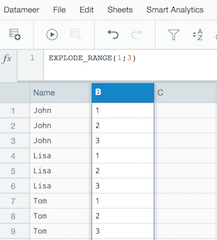•Hello Parth.
I guess the easiest way to achieve this is to use EXPLODE_RANGE function.

• For example - you have 3 names at the Name column.
`JohnLisaTom`
• You would like to repeat every name let's say 3 times, like:
`JohnJohnJohnLisaLisaLisaTomTomTom`
• At the next column introduce the formula EXPLODE_RANGE(1;3) - this will return the next result:• If you need to repeat the name 24 time, the right formula is EXPLODE_RANGE(1;24)

Hope this approach helps.

•If you have 3 name. you can only EXPLODE_RAGE 3 times.

Same like I have 20 names, if I do EXPLODE_RANGE(1;24)

It is still giving me 20 times 1 name.

•Hello Parth.

The function EXPLODE_RANGE will repeat every record at the sheet given number of time. So if you have 20 names (20 records I assume) and introduce the formula EXPLODE_RANGE(1;24), it will make 480 records (20 Names * 24 times).

By default, EXPLODE_RANGE will return only 20 records at the preview mode. You would need to rerun the Workbook to view full results.